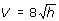Definition Of Formula

A Formula is an expression or equation that expresses the relationship between certain quantities.

For Example, A = pr2 is the formula to find the area of a circle of radius r units.

From this formula, we can know the relationship between the radius and the area of a circle. The area of a circle varies directly as the square of its radius. Here p is the constant of variation.

Solved Example on Formula

Ques: A ball is dropped from a height h ft above the ground. The Formuladescribes the velocity with which the ball reaches the ground in ft/sec. Find V when h = 16 ft.

Choices:

A. 64 ft/sec
B. 128 ft/sec
C. 16 ft/sec
D. 32 ft/sec
Step 1: Substitute h = 16 in the Formula.